Support the creation of content on this website through PATREON!

SEARCHContent by Mika Satomi and Hannah Perner-WilsonThe following institutions have funded our research and supported our work:From 2013-2015 Mika is a guest professor at the eLab at Kunsthochschule Berlin-WeissenseeFrom July - December 2013 Hannah was a researcher at the UdK'sDesign Research Lab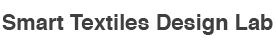From 2010-2012 Mika was a guest researcher in the Smart Textiles Design Lab at The Swedish School of TextilesFrom 2009 - 2011 Hannah was a graduate student in the MIT Media Lab's High-Low Tech research group led by Leah Buechley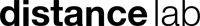In 2009 Hannah and Mika were both research fellows at the Distance Lab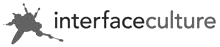Between 2003 - 2009 Hannah and Mika were both students at Interface CulturesWe support the Open Source Hardware movement. All our own designs published on this website are released under the Free Cultural Works definition

The arduino‘s analog pins are reading the voltage that comes in, not the resistance over the two points. When you want to use a resistive sensor (i.e. neoprene bend sensor), you need to manipulate the voltage that goes into the analog pins with the resistive change the sensor makes.

For this, we can make a voltage divider circuit. You can divide the voltage by using 2 resisters. If you have 2 exactly same resistors, the voltage gets half in the middle, like the first diagram. As the ratio between two resisters changes, the voltage you get in the middle (between the resisters) changes accordingly.
One can calculate this by
Supply voltage (5v) x resistanceA / (resistanceA + resistanceB) = divided voltage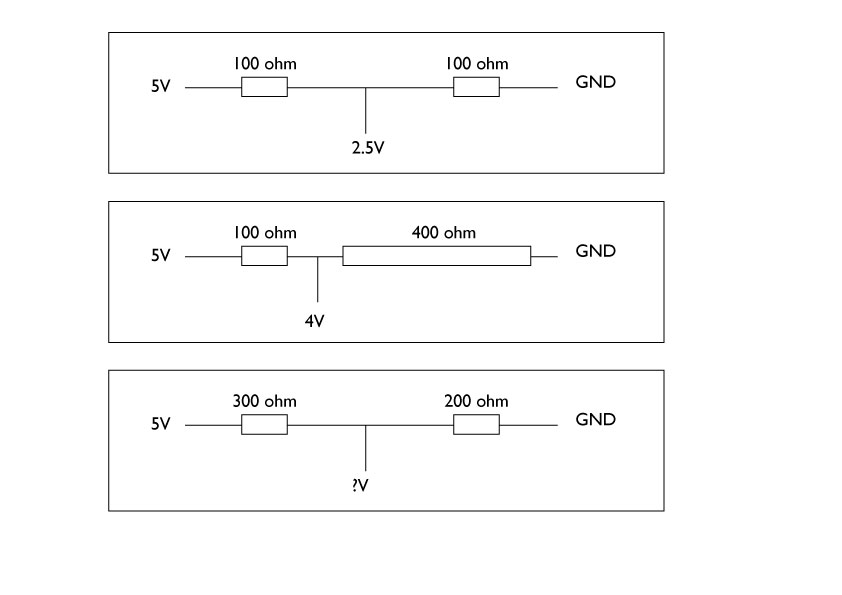So much of a theory, let’s try this to see if it really works. Here is an experiment with two resister with a multumeter.
The first experiment shows two same size resister (10kohm) dividing the provided voltage (5V) in half. The multimeter is set as V– for reading direct current voltage. The probes are connected to 0V (GND) of the power supply and the middle point where two resisters meet. You can see 2.44 in the multilmeter’s display. (almost 2.5V.. maybe the resister had some range) It divides the 5V in 50/50 ratio.In the second experiment, I changed one of the resister to 47kohm. So now the ratio of two resisters are 10/47. So, I should read 5V x 10/(10+47) = 0.877 V in theory. As you can see in multimeter, it is 0.85V it measures. Not bad!Now, if you change one of the resister to our resistive textile sensor, it works the same. The felt sensor I tested here has about 8kohm – 100kohm resistance range. You can see how the voltage that gets divided in the middle changes as I manipulate the felt. Now, if you connect the point where multimeter is reading to the Arduino Analog input, we can read how much voltage comes in.Here is a diagram of how the resistive sensor can be connected with Arduino.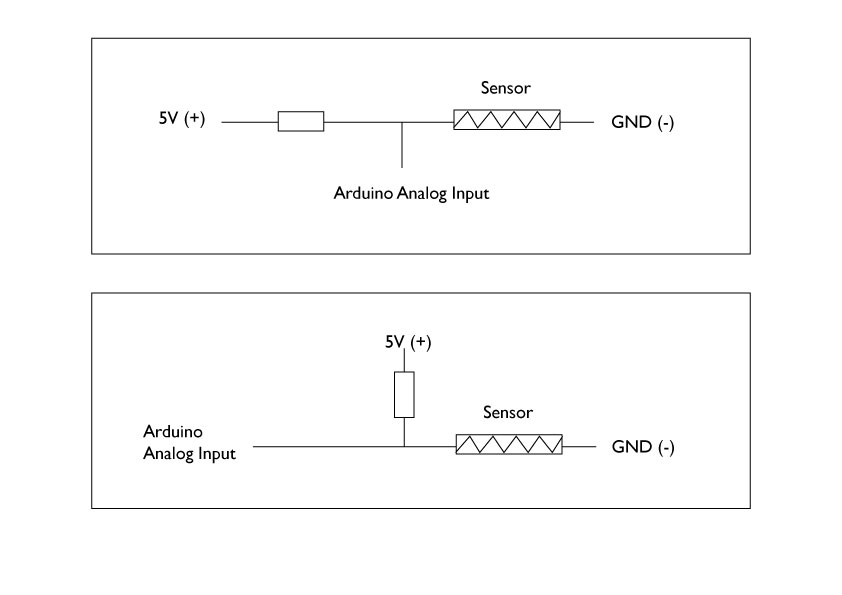The diagram shows the resister on the GND (0V) side switched with the resistive sensor. You can also connect as the supply voltage (5V) side resister as sensor and keep the GND side resister as fixed resister.

Internal Pull Up resister for Analog inputs
In Arduino, it is possible to set “internal pull-up resisters” from the code. The internal pull-up resister size is 20k ohm. When this is set, it is the same as having your selected Analog pin connected to 20k ohm resister that connects to 5V (supply voltage). It is only possible to set as “pull-up” (connected to 5V) and not “pull-down” (connected to ground).
To set these you’ll need to include the following line of code in the setup function of your Arduino sketch. (the example below is for A0 pin)

pinMode(A0, INPUT_PULLUP);